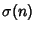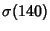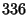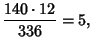## Harmonic Divisor Number

A numberfor which the Harmonic Mean of the Divisors of, i.e.,, is an Integer, whereis the number of Positive integral Divisors ofandis the Divisor Function. For example, the divisors ofare 1, 2, 4, 5, 7, 10, 14, 20, 28, 35, 70, and 140, givingso 140 is a harmonic divisor number. Harmonic divisor numbers are also called Ore Numbers. Garcia (1954) gives the 45 harmonic divisor numbers less than. The first few are 1, 6, 140, 270, 672, 1638, ... (Sloane's A007340).

For distinct Primesand, harmonic divisor numbers are equivalent to Even Perfect Numbers for numbers of the form. Mills (1972) proved that if there exists an Odd Positive harmonic divisor number, thenhas a prime-Power factor greater than.

Another type of number called harmonic'' is the Harmonic Number.

References

Edgar, H. M. W. Harmonic Numbers.'' Amer. Math. Monthly 99, 783-789, 1992.

Garcia, M. On Numbers with Integral Harmonic Mean.'' Amer. Math. Monthly 61, 89-96, 1954.

Guy, R. K. Almost Perfect, Quasi-Perfect, Pseudoperfect, Harmonic, Weird, Multiperfect and Hyperperfect Numbers.'' §B2 in Unsolved Problems in Number Theory, 2nd ed. New York: Springer-Verlag, pp. 45-53, 1994.

Mills, W. H. On a Conjecture of Ore.'' Proceedings of the 1972 Number Theory Conference. University of Colorado, Boulder, pp. 142-146, 1972.

Ore, Ø. On the Averages of the Divisors of a Number.'' Amer. Math. Monthly 55, 615-619, 1948.

Pomerance, C. On a Problem of Ore: Harmonic Numbers.'' Unpublished manuscript, 1973.

Sloane, N. J. A. Sequence A007340/M4299 in An On-Line Version of the Encyclopedia of Integer Sequences.'' http://www.research.att.com/~njas/sequences/eisonline.html and extended entry in Sloane, N. J. A. and Plouffe, S. The Encyclopedia of Integer Sequences. San Diego: Academic Press, 1995.

Zachariou, A. and Zachariou, E. Perfect, Semi-Perfect and Ore Numbers.'' Bull. Soc. Math. Gréce (New Ser.) 13, 12-22, 1972.